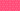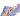PricingResources

# What Are Confidence Intervals?

Written by

When researchers take a sample of the population, there’s always some level of uncertainty involved. No sampling method is perfect, but we can measure this element of uncertainty using confidence intervals. Learn how these measurements work and how to find a confidence interval using our guide below.

## What is a confidence interval?

It’s not a common phrase used outside of statistics, so we’ll start by answering the question: just what is a confidence interval? This term refers to the probability that a range of values will include a population parameter for a specific proportion of times. This proportion is usually expressed as a percentage.

For example, if you have a 95% confidence interval, this represents the range of values in a data set that you could be 95% confident in containing the population’s true mean. A population mean is the average data point between an upper and lower interval.

The confidence interval is important because the mean will vary between samples due to natural variations and unexpected outside influences. If you take multiple samples from the same population, they won’t always be identical. Some samples will show the true population parameter, while others won’t.

When you’re talking about confidence in this context, it means how confident you are in the sampling method itself. In other words, if you repeated the same method multiple times, 95% of the intervals should show the true population mean. The other 5% represents the chance of statistical outliers, or population means sitting outside the upper and lower intervals.

The more samples you take, the more you’re able to narrow down this range and work out a mean with higher statistical accuracy.

## How to find confidence intervals

To find the confidence interval, you’ll need to know the population mean and z-score.

• Mean: Add up the sum of all your numbers, and then divide by the total amount of numbers.

• Z-score: This calculates how far from the mean any data point is, measuring standard deviations above or below the mean.

Z-scores correlate to different confidence levels. The following chart shows how to find confidence intervals using Z-score:

<table> <tbody> <tr> <td> <p><strong><em>Confidence Interval</em></strong></p> </td> <td> <p><em><span style="font-weight: 400;">Z-score</span></em></p> </td> </tr> <tr> <td> <p><span style="font-weight: 400;">90% or 0.90</span></p> </td> <td> <p><span style="font-weight: 400;">1.645</span></p> </td> </tr> <tr> <td> <p><span style="font-weight: 400;">95% or 0.95</span></p> </td> <td> <p><span style="font-weight: 400;">1.96</span></p> </td> </tr> <tr> <td> <p><span style="font-weight: 400;">99% or 0.99</span></p> </td> <td> <p><span style="font-weight: 400;">2.58</span></p> </td> </tr> </tbody> </table>

You’ll then be able to plug in your chosen figures into the confidence interval formula.

Confidence interval formula:

In this formula:

• X = mean

• Z = Z-score

• S = standard error

• N = sample size

So, to calculate the confidence interval scores of a data set, you would divide the standard error by the square root of N. You can then multiply this sum by the Z-score (for example, 1.96 for 95%). The result should be subtracted from the sample mean for a lower interval and added to the sample mean to calculate an upper interval.

This can be complicated on paper, but you can also use programs like Microsoft Excel or online interval calculators to run the numbers for you.

## How to use confidence interval statistics?

Why are confidence interval statistics important, and how are they used in real life? The reason why researchers apply this measurement to data sets is that it accounts for the fact that it’s statistically impossible to study every single member of a population. For research studies, you must select a sub-group or sample. This gives insight into the overall behavior or outcomes of a population, but it won’t account for everyone.

Confidence intervals measure how well the study, or sample, represents your full population. You can increase accuracy, and corresponding confidence intervals, by assessing a higher percentage of the total population. This lets you narrow down means to their truest levels, predicting outliers and yielding more fruitful analysis.

#### We can help

GoCardless helps you automate payment collection, cutting down on the amount of admin your team needs to deal with when chasing invoices. Find out how GoCardless can help you with ad hoc payments or recurring payments.## Interested in automating the way you get paid? GoCardless can help

Contact sales

Sales

Contact Sales

+1(415) 523-2279

Support

help@gocardless.com

+1 (628) 241-0044